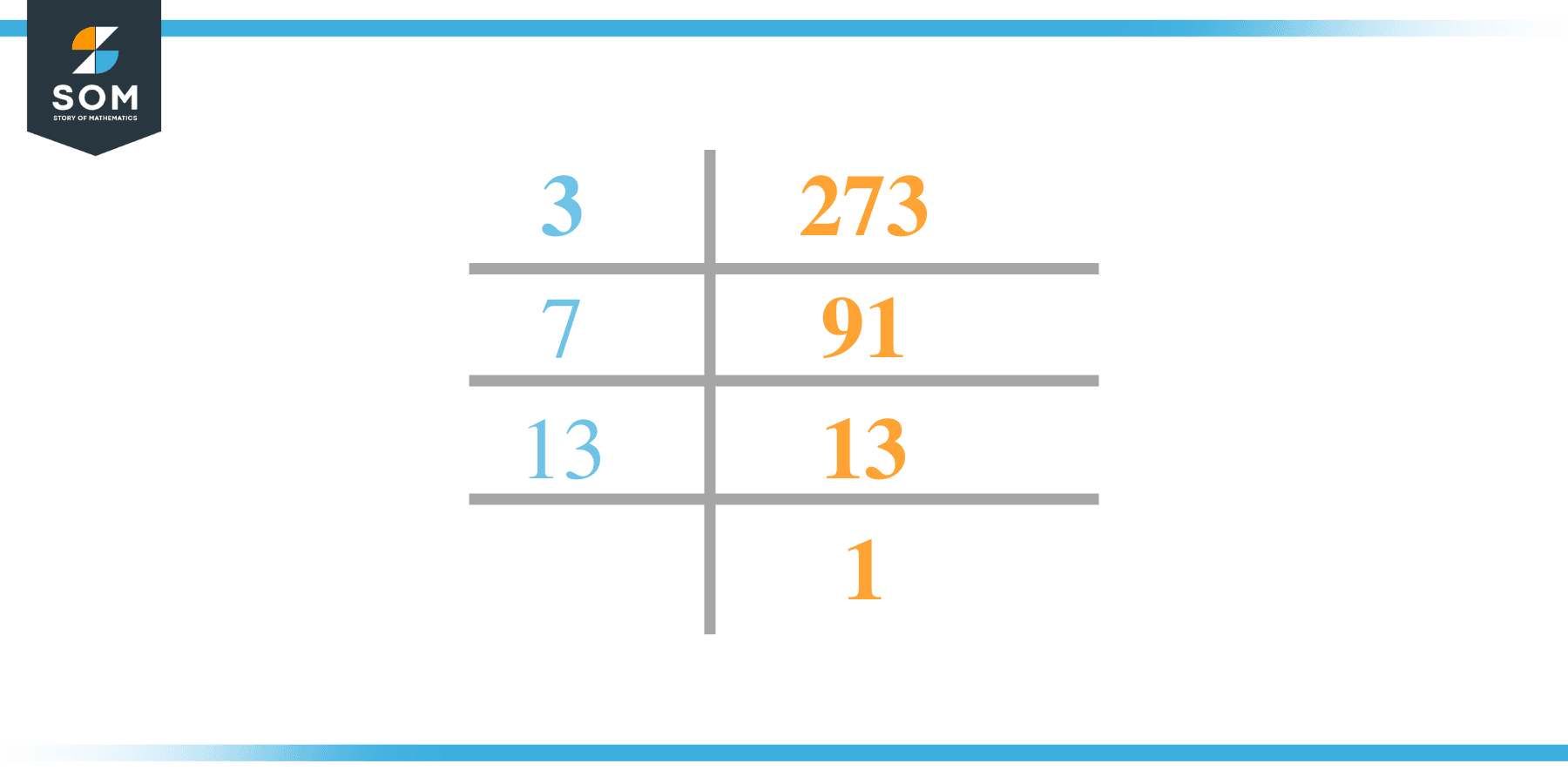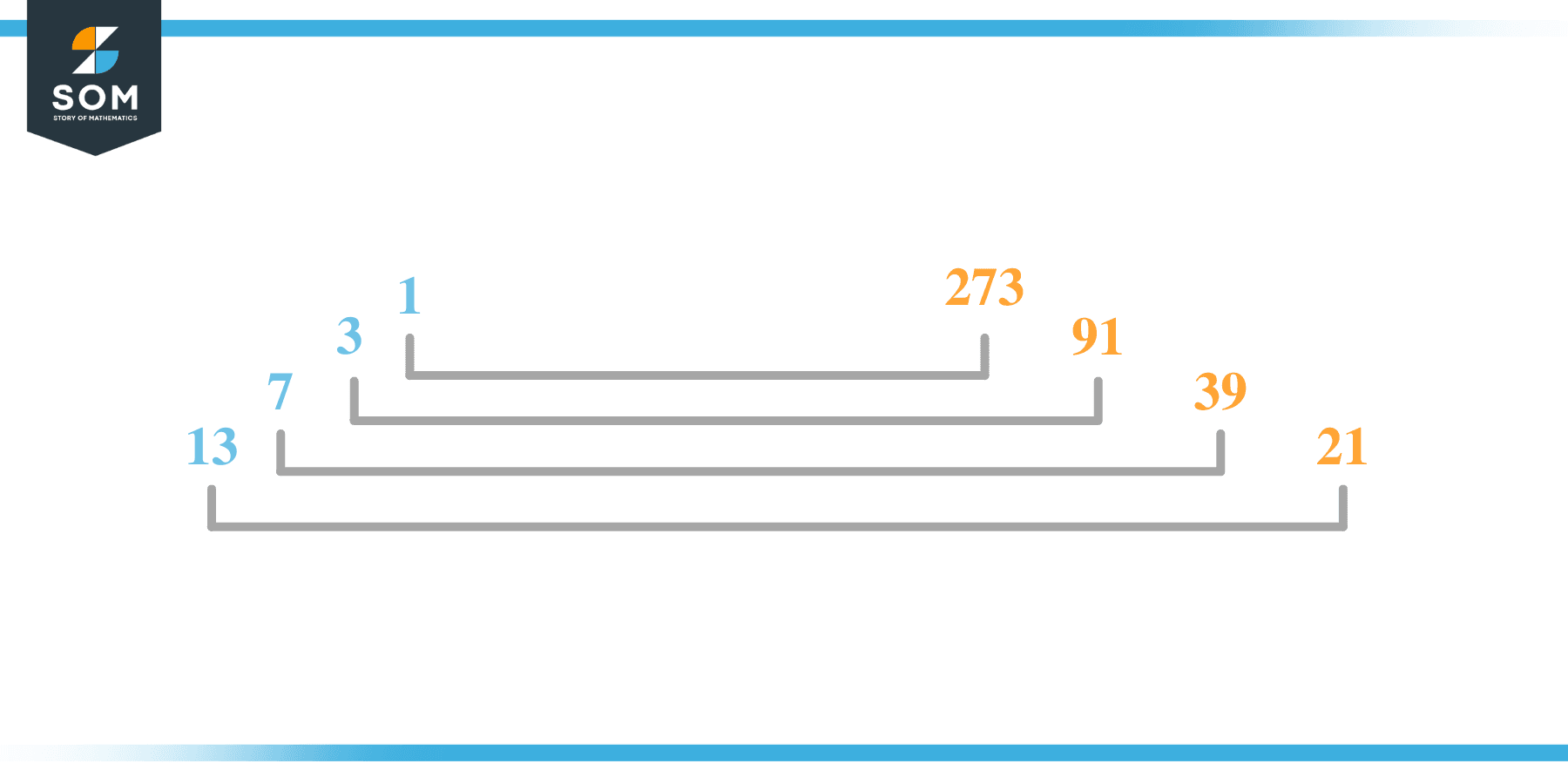# Factors of 273: Prime Factorization, Methods, and Examples

The factors of 273 are the numbers that are divided by 273. 273 is an odd number. It is a composite number as it has more than two factors.The given number’s factors can be positive and negative, provided that the given number is achieved upon multiplication of two-factor integers.

### Factors of 273

Here are the factors of number 273.

Factors of 273: 1, 3, 7, 13, 21, 39, 91, 273

### Negative Factors of 273

The negative factors of 273 are similar to its positive aspects, just with a negative sign.

Negative Factors of 273: –1, -3, -7, -13, -21, -39, -91, -273

### Prime Factorization of 273

The prime factorization of 273 is the way of expressing its prime factors in the product form.

Prime Factorization: 3 x 7 x 13

In this article, we will learn about the factors of 273 and how to find them using various techniques such as upside-down division, prime factorization, and factor tree.

## What Are the Factors of 273?

The factors of 273 are 1, 3, 7, 13, 21, 39, 91, and 273. These numbers are the factors as they do not leave any remainder when divided by 273.

The factors of 273 are classified as prime numbers and composite numbers. The prime factors of the number 273 can be determined using the prime factorization technique.

## How To Find the Factors of 273?

You can find the factors of 273 by using the rules of divisibility. The divisibility rule states that any number, when divided by any other natural number, is said to be divisible by the number if the quotient is the whole number and the resulting remainder is zero.

To find the factors of 273, create a list containing the numbers that are exactly divisible by 273 with zero remainders. One important thing to note is that 1 and 273 are the 273’s factors as every natural number has 1 and the number itself as its factor.

1 is also called the universal factor of every number. The factors of 273 are determined as follows:

$\dfrac{273}{1} = 273$

$\dfrac{273}{3} = 91$

$\dfrac{273}{7} = 39$

$\dfrac{273}{13} = 21$

Therefore, 1, 3, 7, 13, 21, 39, 91, and 273 are the factors of 273.

### Total Number of Factors of 273

For 273, there are 8 positive factors and 8 negative ones. So in total, there are 16 factors of 273.

To find the total number of factors of the given number, follow the procedure mentioned below:

1. Find the factorization/prime factorization of the given number.
2. Demonstrate the prime factorization of the number in the form of exponent form.
3. Add 1 to each of the exponents of the prime factor.
4. Now, multiply the resulting exponents together. This obtained product is equivalent to the total number of factors of the given number.

By following this procedure, the total number of factors of X is given as:

Factorization of 273 is 1 x 3 x 7 x 13.

The exponent of 1, 3, 7, and 13 is 1.

Adding 1 to each and multiplying them together results in 16.

Therefore, the total number of factors of 273 is 16. 8 are positive, and 8 factors are negative.

### Important Notes

Here are some essential points that must be considered while finding the factors of any given number:

• The factor of any given number must be a whole number.
• The factors of the number cannot be in the form of decimals or fractions.
• Factors can be positive as well as negative.
• Negative factors are the additive inverse of the positive factors of a given number.
• The factor of a number cannot be greater than that number.
• Every even number has 2 as its prime factor, the smallest prime factor.

## Factors of 273 by Prime FactorizationThe number 273 is a composite. Prime factorization is a valuable technique for finding the number’s prime factors and expressing the number as the product of its prime factors.

Before finding the factors of 273 using prime factorization, let us find out what prime factors are. Prime factors are the factors of any given number that are only divisible by 1 and themselves.

To start the prime factorization of 273, start dividing by its most minor prime factor. First, determine that the given number is either even or odd. If it is an even number, then 2 will be the smallest prime factor.

Continue splitting the quotient obtained until 1 is received as the quotient. The prime factorization of 273 can be expressed as:

273 = 3 x 7 x 13

## Factors of 273 in PairsThe factor pairs are the duplet of numbers that, when multiplied together, result in the factorized number. Factor pairs can be more than one depending on the total number of factors given.

For 273, the factor pairs can be found as:

1 x 273 = 273

3 x 91 = 273

7 x 39 = 273

13 x 21 = 273

The possible factor pairs of 273 are given as (1, 273),  (3, 91),  (7, 91), and (13, 21 ).

All these numbers in pairs, when multiplied, give 273 as the product.

The negative factor pairs of X are given as:

-1 x -273 = 273

-3 x -91 = 273

-7 x -39 = 273

-13 x -21 = 273

It is important to note that in negative factor pairs, the minus sign has been multiplied by the minus sign, due to which the resulting product is the original positive number. Therefore, -1, -3, -7, -13, -21, -39, 91, and -273 are called negative factors of 273.

## Factors of 273 Solved Examples

To better understand the concept of factors, let’s solve some examples.

### Example 1

How many factors of 273 are there?

### Solution

The total number of Factors of 273 is 8.

Factors of 273 are 1, 3, 7, 13, 21, 39, 91, and 273.

### Example 2

Find the factors of X using prime factorization.

### Solution

The prime factorization of 273 is given as:

273 $\div$ 3 = 91

91 $\div$ 7 = 17

13 $\div$ 13 = 1

So the prime factorization of 273 can be written as:

3 x 7 x 13 = 273